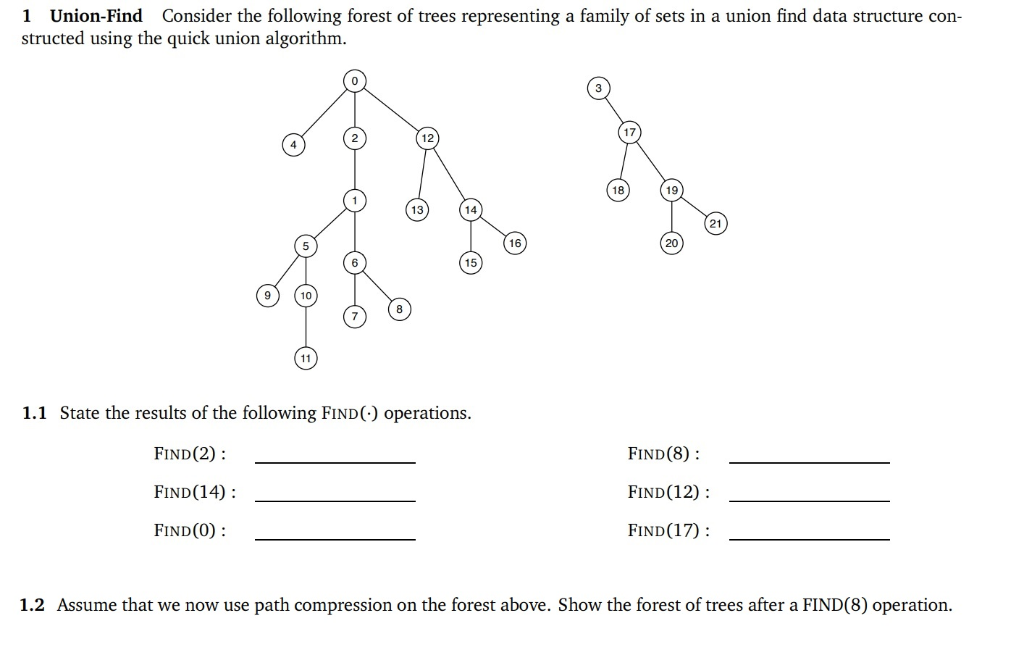# I have 2 questions!

QuestionsCategory: QuestionsI have 2 questions!Before starting the question we will look at how find operation takes place.
int find(int p)
{
while ( p! = id[p] )
p= id[p];
return p;
}
the above given trees are actually stored as an array as below

p
0
1
2
3
4
5
6
7
8
9
10
11
12
13
14
15
16
17
18
19
20
21

id[p]
0
2
0
3
0
1
1
6
6
5
5
10
0
12
12
14
14
3
17
17
19
19

here id[p] is the parent of that node, and if id[p] = p then it means that p is a root
as 0 and 3 are root in our case.
now start to give the answers for the given find operations
1) FIND(2) : as id = 0
: as id = 0
so return 0
2) FIND(8) : as id = 6
: as id = 1
: as id = 2
: as id = 0
: as id = 0
so return 0
3) FIND(14) : as id = 12
: as id = 0
: as id = 0
so return 0
4) FIND(12) : as id = 0
: as id = 0
so return 0
5) FIND(0) : as id = 0
so return 0
6) FIND(17) : as id = 3
: as id = 3
so return 3
Now come to the second part of the question
Now we have to understand the find operation in the path compression method , here is the code for this:
int find(int p)
{
if ( p! = id[p] )
p= find(id[p]);
return p;
}
i.e. when you do find then for every node you traverse just make the root of that tree as parent for that node , so the traversed nodes will get directly connected to the root of that tree .
let’s see the FIND(8) previously
2) FIND(8) : as id = 6
: as id = 1
: as id = 2
: as id = 0
: as id = 0
so return 0
so basically all the nodes 8, 6, 1, 2 will directly connected to the root of the tree i.e. 0 and our table will look like

p
0
1
2
3
4
5
6
7
8
9
10
11
12
13
14
15
16
17
18
19
20
21

id[p]
0
0
0
3
0
1
0
6
0
5
5
10
0
12
12
14
14
3
17
17
19
19
The Tree will look like this: